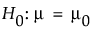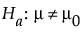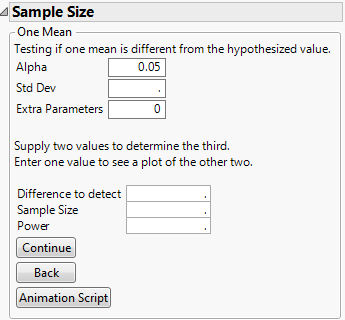Publication date: 06/16/2020

# One Sample Mean Calculator

Use the One Sample Mean calculator to evaluate sample size for a hypothesis test about one mean. Study the trade offs between sample size, power, significance, and the hypothesized difference to detect. Sample size and power are associated with the following hypothesis test:versus the two-sided alternative:where μ is the true mean and μ0 is the null mean or reference value. The difference to detect is an amount, δ, away from μ0 that one considers as important to detect based on a set of samples. For the same significance level and power, a larger sample size is needed to detect a small difference than to detect a large difference. It is assumed that the population of interest is normally distributed with mean μ and standard deviation σ.

Figure 17.3 Initial Sample Size and Power Calculator for One Mean